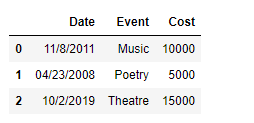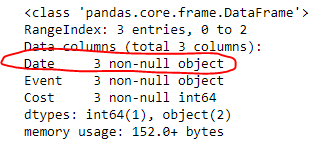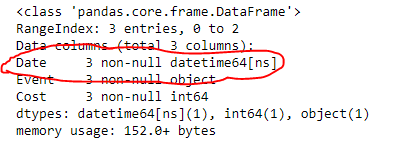# Convert the column type from string to datetime format in Pandas dataframe

While working with data in Pandas, it is not an unusual thing to encounter time series data and we know Pandas is a very useful tool for working with time series data in python.

Let’s see how we can convert a dataframe column of strings (in dd/mm/yyyy format) to datetime format. We cannot perform any time series based operation on the dates if they are not in the right format. In order to be able to work with it, we are required to convert the dates into the datetime format.

Code #1 : Convert Pandas dataframe column type from string to datetime format using `pd.to_datetime()` function.

 `# importing pandas as pd ` `import` `pandas as pd ` ` `  `# Creating the dataframe ` `df ``=` `pd.DataFrame({``'Date'``:[``'11/8/2011'``, ``'04/23/2008'``, ``'10/2/2019'``], ` `                ``'Event'``:[``'Music'``, ``'Poetry'``, ``'Theatre'``], ` `                ``'Cost'``:[``10000``, ``5000``, ``15000``]}) ` ` `  `# Print the dataframe ` `print``(df) ` ` `  `# Now we will check the data type  ` `# of the 'Date' column ` `df.info() `

Output :As we can see in the output, the data type of the ‘Date’ column is object i.e. string. Now we will convert it to datetime format using `pd.to_datetime()` function.

 `# convert the 'Date' column to datetime format ` `df[``'Date'``]``=` `pd.to_datetime(df[``'Date'``]) ` ` `  `# Check the format of 'Date' column ` `df.info() `

Output :As we can see in the output, the format of the ‘Date’ column has been changed to the datetime format.

Code #2 : Convert Pandas dataframe column type from string to datetime format using `DataFrame.astype()` function.

 `# importing pandas as pd ` `import` `pandas as pd ` ` `  `# Creating the dataframe ` `df ``=` `pd.DataFrame({``'Date'``:[``'11/8/2011'``, ``'04/23/2008'``, ``'10/2/2019'``], ` `                ``'Event'``:[``'Music'``, ``'Poetry'``, ``'Theatre'``], ` `                ``'Cost'``:[``10000``, ``5000``, ``15000``]}) ` ` `  `# Print the dataframe ` `print``(df) ` ` `  `# Now we will check the data type ` `# of the 'Date' column ` `df.info() `

Output :As we can see in the output, the data type of the ‘Date’ column is object i.e. string. Now we will convert it to datetime format using `DataFrame.astype()` function.

 `# convert the 'Date' column to datetime format ` `df[``'Date'``] ``=` `df[``'Date'``].astype(``'datetime64[ns]'``) ` ` `  `# Check the format of 'Date' column ` `df.info() `

Output :As we can see in the output, the format of the ‘Date’ column has been changed to the datetime format.

My Personal Notes arrow_drop_upCheck out this Author's contributed articles.

If you like GeeksforGeeks and would like to contribute, you can also write an article using contribute.geeksforgeeks.org or mail your article to contribute@geeksforgeeks.org. See your article appearing on the GeeksforGeeks main page and help other Geeks.

Please Improve this article if you find anything incorrect by clicking on the "Improve Article" button below.

Improved By : Shubham__Ranjan

Article Tags :

4

Please write to us at contribute@geeksforgeeks.org to report any issue with the above content.